# Apply the Pattern to Find the Missing Symbol

In this worksheet, students will find the missing symbol to complete a grid of nine boxes. It will develop their ability to find and follow patterns in shapes and symbols.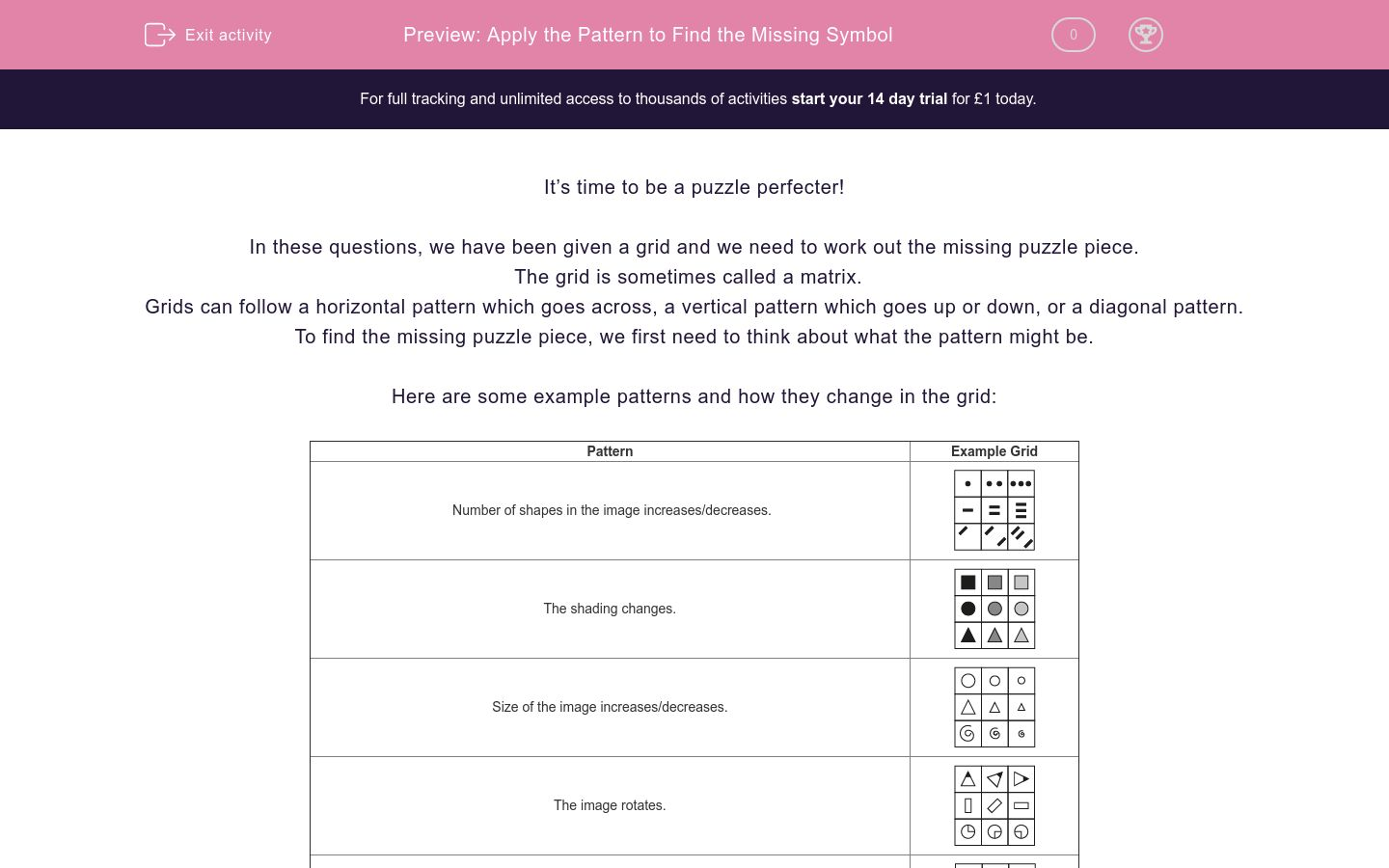Key stage:  KS 2

Curriculum topic:   Spatial and Non-Verbal Reasoning

Curriculum subtopic:   Complete the Grid

Difficulty level:### QUESTION 1 of 10

It’s time to be a puzzle perfecter!

In these questions, we have been given a grid and we need to work out the missing puzzle piece.

The grid is sometimes called a matrix.

Grids can follow a horizontal pattern which goes across, a vertical pattern which goes up or down, or a diagonal pattern.

To find the missing puzzle piece, we first need to think about what the pattern might be.

Here are some example patterns and how they change in the grid:

 Pattern Example Grid Number of shapes in the image increases/decreases.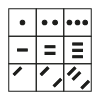The shading changes.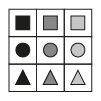Size of the image increases/decreases.The image rotates.Shapes within the symbol change position.The number of sides of the shapes in the image changes.Take a look at this example:Which of the following images would complete this grid?

a)b)c)d)The pattern in this case is horizontal.

The pattern is that the number of sides of each shape increases by one each time. Also, the centre shape gets slightly larger each time. Therefore the answer is b.

It’s now your turn to find the missing puzzle piece in grids. Remember to decide if it is horizontal, vertical or diagonal, when working out what the pattern is.

Good luck puzzle champ!

Always rule out any obviously incorrect answers.

Choose the image from the options below that best completes the grid.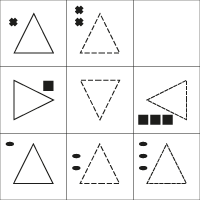a)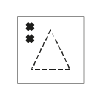b)c)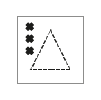d)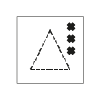e)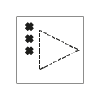Choose the image from the options below that best completes the grid.a)b)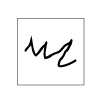c)d)e)Choose the image from the options below that best completes the grid.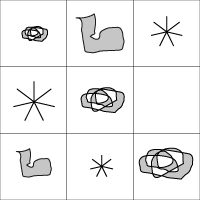a)b)c)d)e)Choose the image from the options below that best completes the grid.a)b)c)d)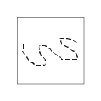e)Choose the image from the options below that best completes the grid.a)b)c)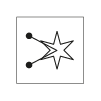d)e)Choose the image from the options below that best completes the grid.a)b)c)d)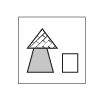e)Choose the image from the options below that best completes the grid.a)b)c)d)e)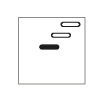Choose the image from the options below that best completes the grid.a)b)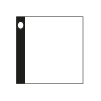c)d)e)Choose the image from the options below that best completes the grid.a)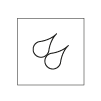b)c)d)e)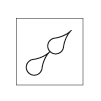Choose the image from the options below that best completes the grid.a)b)c)d)e)• Question 1

Choose the image from the options below that best completes the grid.c)EDDIE SAYS
How did you find the first puzzle detective? Let's start by looking at one shape as a time. Starting with the triangle - did you spot that it had a different outline to the other images? It starts as a full line, then becomes dashed, and in the end column becomes a finer dashed line. This rules out option b as it does not have the correct outline. Now let's look at the orientation of the triangle. Only the middle row has triangles with a different orientation. So we can assume that the missing square in the top row will contain a triangle with the tip pointing upwards, which eliminates option e. Now let's focus on the X shapes in the top left corner of the top row. From left to right, the number of X's is increasing by 1, so we know that the correct answer should have 3 X's. We can also see that they should be in the top left. This means that option c is the best fit in this grid.
• Question 2

Choose the image from the options below that best completes the grid.a)EDDIE SAYS
Working with unfamiliar or irregular shapes is really tricky, so you need to train your detective's eyes to spot similarities and differences in this type, too. We really have to work hard to imagine what they will look like when they are rotated. We can quickly rule out options b and d because they don't fit the pattern because the bottom row contains only the irregular circle shapes. We can see from the top two rows that the middle image is a rotation of the two images on either side of it. Did you spot that the first image has been rotated a half turn (or 180 degrees) to become the central image? Therefore, we can rule out option c as it is exactly the same as the shapes already in this row. So we must choose between option a and option e. The option which shows the shape rotated through 180 degrees or a half turn is option a. Option e is a reflection not a rotation. Make sure you don't get caught out by this puzzle detective!
• Question 3

Choose the image from the options below that best completes the grid.e)EDDIE SAYS
What did you think of the pattern here detective? Did you notice that there were 3 different shapes in each column and row? These shapes are also all different sizes. We can rule out option a straight away because we can already see 3 of this rose shape in the grid. The shape that we are missing is the boot shape. All the shapes have the same orientation in all the squares of the grid. This allows us to find that option e is the best fit in the grid, as it is the only boot which is still upright.
• Question 4

Choose the image from the options below that best completes the grid.d)EDDIE SAYS
In this grid, we are missing the wiggly line with a large dashed outline. This means we can eliminate option b (wrong shape) and option c (wrong thickness) straight away. If we look at the middle and bottom rows, we can see that the image in the middle square is a reflection of the images on either side of it. So is option a, d or e a reflection? The wiggly line of option a is a little shorter so this is not correct. Option e has been reflected upside down, rather than left to right. This means that option d is the correct answer. Well done if you put all those clues together!
• Question 5

Choose the image from the options below that best completes the grid.e)EDDIE SAYS
Pay close attention to the arrowheads here, detective. Did you notice that there are different types? The top row has triangular tips, the middle circles, and the last points. We know that our missing image will have rounded ends, so we can rule out options b and d immediately. In each column there is a star with one line, two lines and three lines coming from it. If we count the totals of each in the grid, we can see that the missing image must be a star with three lines coming from it. This eliminates option c. So we are left with a choice between option a and e. We can see that in all of the images in the grid, the lines coming from the stars are evenly spaced. In option a they are not evenly spaced, so this cannot be the best fit. Option e is the best fit in this grid as it has three lines, with rounded ends, which are evenly spaced. Phew! Well done if you got that one detective!
• Question 6

Choose the image from the options below that best completes the grid.c)EDDIE SAYS
Good try puzzle detective! In each of the squares of this grid, there are three shapes with different colours. So we know that the missing image will involve the same collection of objects or shapes as the images on either side of it. The objects stay in the same positions, so we can rule out option d immediately. We can also rule out option e, because there is no black element in the other images in this row. Similarly, option a contains different shading (the checked roof) which also doesn't appear in this row. So we have to choose between options b and c. How do we know which would be the best fit? The clue is in the rectangle to the left of the arrow house. The three colour options used in the row are white, grey and diagonal bricks. We can see that we have used the diagonal bricks and grey on the rectangle in the other images, so the missing image must have a white rectangle. This makes option c the best fit in this grid. That was a superb piece of detective work if you solved that puzzle.
• Question 7

Choose the image from the options below that best completes the grid.b)EDDIE SAYS
We are looking for 4 lines to complete the pattern in this grid, so we can rule out option e from the start. The squares above and below the missing square contain one black shape in the group of four, so we can also eliminate option c. Now we need to look at how the remaining options fit in the grid. Option d matches the images in the left-hand column, but does it fit with the rest of the row? The other two squares in the row start from the top right corner so this is not a good fit. The best fit to replicate this pattern is, therefore, option b. Great working following all those STEPS to the correct answer!
• Question 8

Choose the image from the options below that best completes the grid.c)EDDIE SAYS
Sometimes the images that seem simple can be the hardest to work out. Remember to go back to the basics and say what you see. We can see that the first column has a white circle and no lines, the second column has one circle and one black line, and the third column has one circle and two black lines. As the missing image has one black line this rules out option e. Now let's look at the position of the circles and lines. In the middle row, the circles are positioned in the centre of the grid square, so we can eliminate options a and b as they do not follow this pattern. Now where should the black line be? In the far right-hand column of boxes, the black line is on the left-hand side and middle. Therefore, we can assume that in the missing square the black line will be in the middle, which makes option c the best fit.
• Question 9

Choose the image from the options below that best completes the grid.b)EDDIE SAYS
This one is similar to others you may have seen and like a sudoku puzzle. We need to have 1, 2 and 3 images in every row and column. If we look at the groups of images, we can see that we are missing an image with 2 suns, so we can choose between options b, c and d. Now let's look at the alignment of the suns. In all the sun-themed squares of the grid, they are aligned to the top, left corner. This means that option b is the best fit in this grid. Just one question to go grid superstar!
• Question 10

Choose the image from the options below that best completes the grid.a)EDDIE SAYS
This question is testing your ability to visualise angles, another top detective skill! Look carefully at the shapes. What rotation are they going through? Did you spot that they are turning through 45 degrees? If you can't see this easily, then get a protractor to help you with this one. If we rotate the image to the left of the blank space by 45 degrees, it will end up like option a which is why this is the best fit. Remember that we are not looking for just a good fit, we are looking for the BEST fit. Fantastic work detective! You've now completed this activity.
---- OR ----

Sign up for a £1 trial so you can track and measure your child's progress on this activity.

### What is EdPlace?

We're your National Curriculum aligned online education content provider helping each child succeed in English, maths and science from year 1 to GCSE. With an EdPlace account you’ll be able to track and measure progress, helping each child achieve their best. We build confidence and attainment by personalising each child’s learning at a level that suits them.

Get started